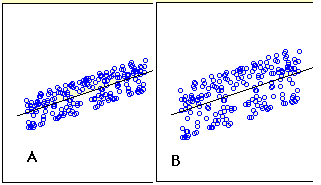# 14.4: Standard Error of the Estimate

•• David Lane
• Rice University
$$\newcommand{\vecs}{\overset { \rightharpoonup} {\mathbf{#1}} }$$ $$\newcommand{\vecd}{\overset{-\!-\!\rightharpoonup}{\vphantom{a}\smash {#1}}}$$$$\newcommand{\id}{\mathrm{id}}$$ $$\newcommand{\Span}{\mathrm{span}}$$ $$\newcommand{\kernel}{\mathrm{null}\,}$$ $$\newcommand{\range}{\mathrm{range}\,}$$ $$\newcommand{\RealPart}{\mathrm{Re}}$$ $$\newcommand{\ImaginaryPart}{\mathrm{Im}}$$ $$\newcommand{\Argument}{\mathrm{Arg}}$$ $$\newcommand{\norm}{\| #1 \|}$$ $$\newcommand{\inner}{\langle #1, #2 \rangle}$$ $$\newcommand{\Span}{\mathrm{span}}$$ $$\newcommand{\id}{\mathrm{id}}$$ $$\newcommand{\Span}{\mathrm{span}}$$ $$\newcommand{\kernel}{\mathrm{null}\,}$$ $$\newcommand{\range}{\mathrm{range}\,}$$ $$\newcommand{\RealPart}{\mathrm{Re}}$$ $$\newcommand{\ImaginaryPart}{\mathrm{Im}}$$ $$\newcommand{\Argument}{\mathrm{Arg}}$$ $$\newcommand{\norm}{\| #1 \|}$$ $$\newcommand{\inner}{\langle #1, #2 \rangle}$$ $$\newcommand{\Span}{\mathrm{span}}$$

Learning Objectives

• Make judgments about the size of the standard error of the estimate from a scatter plot
• Compute the standard error of the estimate based on errors of prediction
• Compute the standard error using Pearson's correlation
• Estimate the standard error of the estimate based on a sample

Figure $$\PageIndex{1}$$ shows two regression examples. You can see that in $$\text{Graph A}$$, the points are closer to the line than they are in $$\text{Graph B}$$. Therefore, the predictions in $$\text{Graph A}$$ are more accurate than in $$\text{Graph B}$$.Figure $$\PageIndex{1}$$: Regressions differing in accuracy of prediction

The standard error of the estimate is a measure of the accuracy of predictions. Recall that the regression line is the line that minimizes the sum of squared deviations of prediction (also called the sum of squares error). The standard error of the estimate is closely related to this quantity and is defined below:

$\sigma _{est}=\sqrt{\frac{\sum (Y-Y')^2}{N}}$

where $$\sigma _{est}$$ is the standard error of the estimate, $$Y$$ is an actual score, $$Y'$$ is a predicted score, and $$N$$ is the number of pairs of scores. The numerator is the sum of squared differences between the actual scores and the predicted scores.

Note the similarity of the formula for $$\sigma _{est}$$ to the formula for σ. ￼ It turns out that σest is the standard deviation of the errors of prediction (each $$Y - Y'$$ is an error of prediction).

Assume the data in Table $$\PageIndex{1}$$ are the data from a population of five $$X$$, $$Y$$ pairs.

Table $$\PageIndex{1}$$: Example data
X Y Y' Y-Y' (Y-Y')2
1.00 1.00 1.210 -0.210 0.044
2.00 2.00 1.635 0.365 0.133
3.00 1.30 2.060 -0.760 0.578
4.00 3.75 2.485 1.265 1.600
5.00 2.25 2.910 -0.660 0.436
Sum 15.00 10.30 10.30 0.000 2.791

The last column shows that the sum of the squared errors of prediction is $$2.791$$. Therefore, the standard error of the estimate is

$\sigma _{est}=\sqrt{\frac{2.791}{5}}=0.747$

There is a version of the formula for the standard error in terms of Pearson's correlation:

$\sigma _{est}=\sqrt{\frac{(1-\rho )^2SSY}{N}}$

where $$ρ$$ is the population value of Pearson's correlation and $$SSY$$ is

$SSY=\sum (Y-\mu _Y)^2$

For the data in Table $$\PageIndex{1}$$, $$μ_Y = 2.06$$, $$SSY = 4.597$$ and $$ρ= 0.6268$$. Therefore,

$\sigma _{est}=\sqrt{\frac{(1-0.6268^2)(4.597)}{5}}=\sqrt{\frac{2.791}{5}}=0.747$

which is the same value computed previously.

Similar formulas are used when the standard error of the estimate is computed from a sample rather than a population. The only difference is that the denominator is $$N-2$$ rather than $$N$$. The reason $$N-2$$ is used rather than $$N-1$$ is that two parameters (the slope and the intercept) were estimated in order to estimate the sum of squares. Formulas for a sample comparable to the ones for a population are shown below.

$s _{est}=\sqrt{\frac{\sum (Y-Y')^2}{N-2}}$

$s _{est}=\sqrt{\frac{2.791}{3}}=0.964$

$s _{est}=\sqrt{\frac{(1-r)^2SSY}{N-2}}$

This page titled 14.4: Standard Error of the Estimate is shared under a Public Domain license and was authored, remixed, and/or curated by David Lane via source content that was edited to the style and standards of the LibreTexts platform; a detailed edit history is available upon request.